CAT  >  Test: Algebra - 1

# Test: Algebra - 1

Test Description

## 30 Questions MCQ Test Topic-wise Past Year Questions for CAT | Test: Algebra - 1

Test: Algebra - 1 for CAT 2022 is part of Topic-wise Past Year Questions for CAT preparation. The Test: Algebra - 1 questions and answers have been prepared according to the CAT exam syllabus.The Test: Algebra - 1 MCQs are made for CAT 2022 Exam. Find important definitions, questions, notes, meanings, examples, exercises, MCQs and online tests for Test: Algebra - 1 below.
Solutions of Test: Algebra - 1 questions in English are available as part of our Topic-wise Past Year Questions for CAT for CAT & Test: Algebra - 1 solutions in Hindi for Topic-wise Past Year Questions for CAT course. Download more important topics, notes, lectures and mock test series for CAT Exam by signing up for free. Attempt Test: Algebra - 1 | 30 questions in 50 minutes | Mock test for CAT preparation | Free important questions MCQ to study Topic-wise Past Year Questions for CAT for CAT Exam | Download free PDF with solutions
 1 Crore+ students have signed up on EduRev. Have you?
Test: Algebra - 1 - Question 1

### If a1 + a2 + a3 + ... + an = 3(2n+1 – 2), for every n ≥ 1, then a11 equals (2019)

Detailed Solution for Test: Algebra - 1 - Question 1

If n = 1, then a1 = 3(21 + 1 – 2) = 6 = 3 × 21
If n =  2, then a1 + a2 = 3(22 + 1 – 2) = 18 ⇒ a2
= 18 – a1 = 18 – 6 = 12 = 3 × 22
If n = 3, then a1 + a2 + a3 = 3(23 + 1 – 2) = 42 ⇒ a3
= 42 – (a1 + a2) = 24 = 3 × 23
Similarly, an = 3 × 2n
Therefore, a11 = 3 × 211 = 6144

Test: Algebra - 1 - Question 2

### The number of solutions to the equation |x|(6x2 + 1) = 5x2 is (2019)

Detailed Solution for Test: Algebra - 1 - Question 2

Case I: x = 0.
Clearly, x = 0 satisfy the equation
Case II: x > 0 |x| (6x2 + 1) = 5x2 ⇒ x(6x2 + 1) = 5x2
⇒ x(6x2 + 1 – 5x) = 0
∴ x ≠ 0
∴ 6x2 + 1 – 5x = 0
On solving the quadratic equation, we get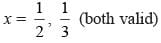Case III: x < 0
|x| (6x2 + 1) = 5x2 ⇒ –x(6x2 + 1) = 5x2
⇒ –x(6x2 + 1 + 5x) = 0
⇒ x(6x2 + 5x + 1) = 0
∵ x ≠ 0, ∴ 6x2 + 5x + 1 = 0
On solving the quadratic equation, we get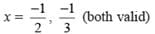Hence there are 5 solutions.

Test: Algebra - 1 - Question 3

### The product of the distinct roots of |x2 – x – 6| = x + 2 is (2019)

Detailed Solution for Test: Algebra - 1 - Question 3

x2 – x – 6 = (x + 2)(x – 3)
Case 1: x2 – x – 6 < 0 ⇒ (x + 2)(x – 3) < 0
⇒ –2 < x < 3 and |x2 – x – 6| = –(x2 – x – 6)
∴ |x2 – x – 6| = x + 2 ⇒ –(x + 2)(x – 3) = x + 2
⇒ (x – 3) = –1 ⇒ x = 2
Case 2: x2 – x – 6 ≥ 0 ⇒ (x + 2) (x – 3) ≥ 0
⇒ x ≤ –2 or x ≥ 3
Checking for boundary conditions:
For x = –2, |x2 – x – 6| = x + 2, therefore,x = -2 is also the root.
But for x = 3, |x2 – x – 6| ≠ x + 2
Hence x = 3 is NOT the root.
And for the interval x < –2 or x > 3 the expression |x2 – x – 6|
= x2 – x – 6
∴ |x2 – x – 6| = x + 2
⇒ (x + 2)(x – 3) = x + 2
⇒ (x – 3) = 1 ⇒ x = 4
Hence, the root are –2, 2, and 4.
∴ Required product = (2)(–2)(4) = –16

Test: Algebra - 1 - Question 4

The number of the real roots of the equation 2cos (x(x + 1)) = 2x + 2–x is

(2019)

Detailed Solution for Test: Algebra - 1 - Question 4

Given: 2 cos (x(x + 1)) = 2x + 2–x
Now, by AM – GM inequality, we get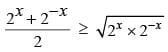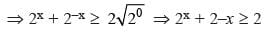∴ 2 cos (x(x + 1)) ≥ 2
We know, –1 ≤ cosθ ≤ 1
∴ 2 cos(x(x + 1)) = 2
Hence, the expression is valid only if 2x + 2–x = 2, which is true for only one value of x i.e. 0.
Therefore, the expression has only one real solution.

Test: Algebra - 1 - Question 5

For any positive integer n, let f(n) = n(n + 1) if n is even, and f(n) = n + 3 if n is odd. If m is a positive integer such that 8f(m + 1) – f(m) = 2, then m equals

(2019)

Detailed Solution for Test: Algebra - 1 - Question 5

Case 1: m is even
Given, 8f(m + 1) – f(m) = 2
⇒ 8(m + 1 + 3) – m(m + 1) = 2
⇒ 8m + 32 – m2 – m = 2
⇒ m2 – 7m – 30 = 0
⇒ (m – 10)(m + 3) = 0
⇒ m = 10 or –3
As m is a positive integer, therefore m = 10.
Case 2: If m is odd, then
8f(m + 1) – f(m) = 2
⇒ 8(m + 1)(m + 2) – (m + 3) = 2
⇒ 8(m2 + 3m +  2) – m – 3 = 2
⇒ 8m2 + 24m + 16 – m – 3 = 2
⇒ 8m2 + 23m + 11 = 0,
Which is not possible and hence no solution.

Test: Algebra - 1 - Question 6

Consider a function f satisfying f (x + y) = f (x) f (y) where x, y are positive integers, and f (1) = 2. If f (a + 1) + f (a + 2) + ... + f (a + n) = 16(2n – 1) then a is equal to

(2019)

Detailed Solution for Test: Algebra - 1 - Question 6

Given,
f(a + 1) + f(a + 2) + .. + f(a + n) = 16(2n – 1)
⇒ f(a) f(1) + f(a) f(2) + ... + f(a) f(n) = 16(2n – 1)
⇒ f(a) (f(1) + f(2) + ... + f(n)) = 16 (2n – 1)
When n = 1, then f(a)f(1) = 16(21 – 1) = 16
⇒ f(a) × 2 = 16 ⇒ f(a) = 8
∴ f(a) (f(1) + f(2) + ... + f(n)) = 16(2n – 1)
⇒ f(1) + f(2) + ... + f(n) = 2(2n – 1)
When n = 2, then f(1) + f(2) = 2(22 – 1) = 6
⇒ f(2) = 6 – f(1) = 6 – 2 = 4
When n = 3, then f(1) + f(2) + f(3) = 2(23 – 1) = 14
⇒ f(3) = 14 – f(1) – f(2) = 14 – 2 – 4 = 8 = f(a)
∴ a = 3

Test: Algebra - 1 - Question 7

The real root of the equation 26x + 23x+2 – 21 = 0 is

(2019)

Detailed Solution for Test: Algebra - 1 - Question 7

26x + 23x+2 – 21 = 0
⇒ 26x + 23x × 22 – 21 = 0
Put 23x = y, then y2 + 4y – 21 = 0
⇒ (y – 3) (y + 7) = 0 ⇒ y = 3 or y = –7
⇒ 23x = 3 or 23x = –7 (No solution)
⇒ 3x = log23 ⇒ x = log2 3 / 3

Test: Algebra - 1 - Question 8

What is the largest positive integer n such that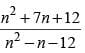is also a positive integer?

(2019)

Detailed Solution for Test: Algebra - 1 - Question 8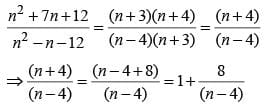The expression is positive integer if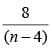is non-negative integer.
For this (n – 4) must be factor of 8.
For n to be largest, n – 4 = 8
∴ n = 12

Test: Algebra - 1 - Question 9

If 5x – 3y = 13438 and 5x – 1 + 3y + 1 = 9686, then x + y equals

(2019)

Detailed Solution for Test: Algebra - 1 - Question 9

Consider the second given equation
5x–1 + 3y+1 = 9686 ...(i)
Last digit of 5x–1 will always be 5 for all positive integral values of (x – 1)
The power cycle of 3 is
34k+1 ≡ 3
34k+2 ≡ 9
34k+3 ≡ 7
34k ≡ 1
Clearly 3y+1 must be in the form of 34k as the unit digit of
R.H.S. = 6 of eq. (i)
We have 34 = 81, and 38 = 6561
Also, 9686 – 81 = 9605 and 9686 – 6561 = 3125
We observe that 3125 = 55
∴ 5x–1 = 55 ⇒ x = 6
and 3y+1 = 38 ⇒ y = 7
x = 6 and y = 7 also satisfies the first given equation 5x – 3y = 13438
∴ x + y = 6 + 7 = 13

Test: Algebra - 1 - Question 10

The quadratic equation x2 + bx + c = 0 has two roots 4a and 3a, where a is an integer. Which of the following is a possible value of b2 + c?

(2019)

Detailed Solution for Test: Algebra - 1 - Question 10

Sum of roots = 4a + 3a = 7a = –b
∴ b = –7a
Product of roots = 4a × 3a = c
∴ c = 12a2
Now, b2 +  c = (–7a)2 + 12a2 = 61a2
Comparing the options
Option (a) : 61a2 = 3721 ⇒ a2 = 61, clearly a is not an integer.
Option (b) : 61a2 = 361 ⇒ a2 = 361 / 61, clearly a is not an integer.
Option (c) : 61a2 = 549 ⇒ a2 = 9, we can have a = –3 or 3 (an integer)
Option (d) : 61a2 = 427 ⇒ a2 = 7, clearly a is not an integer

Test: Algebra - 1 - Question 11

Let a, b, x, y be real numbers such that a2 + b2 = 25, x2 + y2 = 169, and ax + by = 65. If k = ay – bx, then

(2019)

Detailed Solution for Test: Algebra - 1 - Question 11

We can take a = 5, b = 0, x = 13 and y = 0 as these values that satisfies all three equations
∴ k = ay – bx = 5 × 0 – 0 × 13 = 0

Test: Algebra - 1 - Question 12

If u2 + (u − 2v − 1)2 = −4v(u + v), then what is the value of u + 3v?

(2018)

Detailed Solution for Test: Algebra - 1 - Question 12

Cost of table of Amal = 1.2p
Cost of table for Asim = 0.8p
Amal sells to Bimal at 1.3 × 1.2p = 1.56p
= cost of table for Bimal = x
Asim sells table to Barun at 0.7 × 0.8p = 0.56p
= cost of table for Barun = y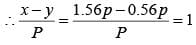Test: Algebra - 1 - Question 13

If f(x) = 5x + 2 / 3x - 5 and g(x) = x2 – 2x – 1, then the value of g (f(f(3))) is

(2017)

Detailed Solution for Test: Algebra - 1 - Question 13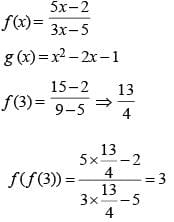g (f(f(3))) = (3)2 – 3 × 2 – 1 = 9 – 6 – 1 = 9 – 7 = 2

Test: Algebra - 1 - Question 14

The number of solutions (x, y, z) to the equation x – y – z = 25, where x, y, and z are positive integers such that x ≤ 40, y ≤ 12,and z ≤ 12 is

(2017)

Detailed Solution for Test: Algebra - 1 - Question 14

x = 25 + y + z
Possible value table below.
27 ≤ x ≤ 40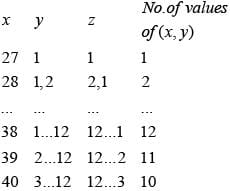The no. of solution is 1 + 2 + ... + 12 + 11 + 10 = 99

Test: Algebra - 1 - Question 15

Suppose, log3 x = log12 y = a, where x, y are positive numbers. If G is the geometric mean of x and y, and log6 G is equal to

(2017)

Detailed Solution for Test: Algebra - 1 - Question 15

log3x = a, x = 3a
log12y = a, y = 12a
Therefore, the geometric mean of x and y equals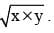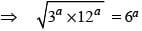Hence, 6a or log6 G = a

Test: Algebra - 1 - Question 16

If the sum to infinity of the series 2 + (2 – d) 2/3 + (2 + d) 4/9 + (2 + 3d) 8/27 + .... is 5/2, what is the value of d?

(2016)

Detailed Solution for Test: Algebra - 1 - Question 16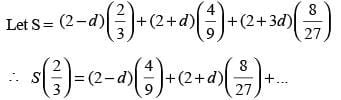Subtracting,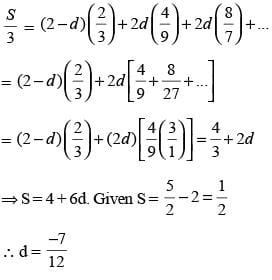Test: Algebra - 1 - Question 17

If S = 2/10 + 6/102 + 12/103 + 20/104 + 30/105 + 42/106 + ......., find the value of S?

(2016)

Detailed Solution for Test: Algebra - 1 - Question 17

∵ S = 0.2 + 0.06 + 0.012 + 0.0020 + 0.00030 + 0.000042 + ...
⇒ S = 0.274342 + ...............
Going from answer choices, choice (a) = 0.2666 ....
Choice (b) = 0.268888 .....; choice (c) = 0.27222 ...
Choice (d) = 0.27434 ...

Test: Algebra - 1 - Question 18

If the roots of the equation (x + 1) (x + 9) + 8 = 0 are a and b, then the roots of the equation (x + a) (x + b) – 8 = 0 are

(2016)

Detailed Solution for Test: Algebra - 1 - Question 18

Here, (x + 1) (x + 9) + 8 = 0
x2 + 10x + 17 = 0
The roots of the equation are a and b
∴ a + b = – 10
ab = 17
(x + a) (x + b) – 8 = 0
x2 + (a + b) x + ab – 8 = 0
x2 – 10x  +  9 = 0
Therefore, roots of (x + a) (x + b) – 8 = 0 are 1 and 9.

Test: Algebra - 1 - Question 19

If x2 + (x + 1) (x + 2) (x + 3) (x + 6) = 0, where x is a real number, then one value of x that satisfies this equation is

(2015)

Detailed Solution for Test: Algebra - 1 - Question 19

x2 + ( x + 1) (x + 2) (x + 3) (x + 6) = 0
(x + 1) (x + 2) (x + 3) (x + 6) = –x2
(x2 + 7x + 6) (x2  + 5x + 6) = –x2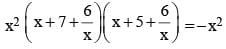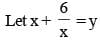then, (y + 7) (y + 5) = –1
⇒ y2 + 12y + 35 = –1
y2 + 12y + 36 = 0
⇒ (y + 6)2  = 0
⇒ y = – 6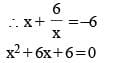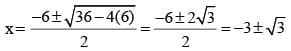Test: Algebra - 1 - Question 20

Find the solution set for [x] + [2x] + [3x] = 8, where x is a real number and [x] is the greatest integer less than or equal to x.

(2015)

Detailed Solution for Test: Algebra - 1 - Question 20

By observing we can find that x > 1 and x < 2.
Else the RHS ≠ 8.
So the combinations are [x] = 1, [2x] = 2 or 3.
[3×] = 4 or 5
The combinations that give RHS = 8 are 1 + 2 + 5 or 1 + 3 + 4.
For any value of x, the case of "1 + 2 + 5" is not possible. Hence it has to be the case of "1 + 3 + 4".
Which will occur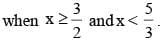Hence the solution is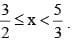Test: Algebra - 1 - Question 21

If 3x + y + 4 = 2xy, where x and y are natural numbers, then find the ratio of the sum of all possible values of x to the sum of all possible values of y.

(2015)

Detailed Solution for Test: Algebra - 1 - Question 21

3x + y + 4 = 2x y
⇒ 3x + 4 = y (2x – 1)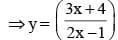When x = 6 ⇒ y = 2
When x = 1 ⇒ y = 7
These two are the only possible pairs of values of x and y. Where x and y are natural numbers.
∴  Required ratio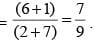Test: Algebra - 1 - Question 22

Let x, y, z and t be the positive numbers which satisfy the following conditions:
I. If x > y, then z > t and
II. If x > z, then y < t
Which of the following is necessarily true?

(2015)

Detailed Solution for Test: Algebra - 1 - Question 22

By assuming the values of x, y, z and t, (a) and (b) can be very easily ruled out.
Checking option (c), if x > y + z. then x > y and x > z (since all numbers are positive).
So, using statements I and II, x > z > t > y.
So, option (c) is correct.

Test: Algebra - 1 - Question 23

Both the roots of the quadratic equation x2 + rx + s = 0 are real and greater than 1. If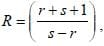then which of the following is definitely true?

(2015)

Detailed Solution for Test: Algebra - 1 - Question 23

Take roots as 2, 2
⇒ r = – 4 & s = 4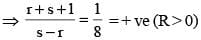Test: Algebra - 1 - Question 24

If x(x – 3) = –1, then the value of x3(x3 – 18) is

(2014)

Detailed Solution for Test: Algebra - 1 - Question 24

According to question,
x (x – 3) = –1
cubing on both side.
⇒ x3 (x – 3)3 = (–1)3
⇒ x3 (x3– 27 – 9x2 + 27x) = – 1
⇒ x3 (x3 – 18) + x3 (–9 – 9x2 + 27x) = –1
⇒ x3 (x3 – 18) – 9x3 (x2 – 3x + 1) = –1
⇒ x3 (x3 – 18) – 9x3 (–1 + 1) = –1
⇒ x3 (x3 – 18) = –1

Test: Algebra - 1 - Question 25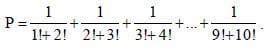Find the value of P.

(2014)

Detailed Solution for Test: Algebra - 1 - Question 25

nth term of the series can be written as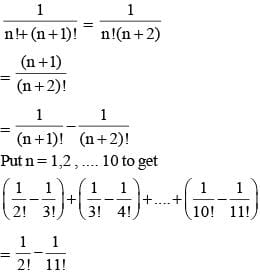Test: Algebra - 1 - Question 26

What is the sum of the roots of all the quadratic equations that can be formed such that both the roots of the quadratic equation are common with the roots of equation (x – a) (x – b) (x – c) = 0?

(2014)

Detailed Solution for Test: Algebra - 1 - Question 26

The equations formed by the roots of the equation
(x – a) (x – b)(x – c) can be as follows:
(i)  (x – a)(x – b) ⇒ Roots are a, b
(ii) (x – b)(x – c) ⇒ Roots are b, c
(iii) (x – c)(x – a) ⇒ Roots are c, a
(iv) (x – a)2 ⇒ Roots are a, a
(v) (x – b)2 ⇒ Roots are b, b
(vi) (x – c)2 ⇒ Roots are c, c
Adding all these roots, we get 4(a + b + c).

Test: Algebra - 1 - Question 27

The sum of the coefficients of the polynomial (x – 1)9 (x – 2)4 (x – 4) is

(2014)

Detailed Solution for Test: Algebra - 1 - Question 27

We obtain the sum of all the coefficients of a polynomial by equating all the variables to 1. Here by putting x = 1 in the polynomial, the required sum comes out to be zero.

Test: Algebra - 1 - Question 28

An equation with all positive roots is written as Xn + anXn-1 + an-1Xn-2 + .... a1 = 0. Which of the following in necessarily true?

(2014)

Detailed Solution for Test: Algebra - 1 - Question 28

Here, (x – 1) (x  – 2) (x – 3) = 0
⇒ (x2 – 3x + 2) (x – 3) = 0
⇒ x3 – 6x2 + 11x – 6 = 0
According to question,
x = 3, an = –6, a1 = –6
Substitute the above values in the option,
Option (a) : (–6)3 ≥ 33 × – 6
⇒ – 216 ≥ – 168
This is incorrect, thus option (a) is incorrect.
Opiton (b) : 33 ≥ (–6)3 × –6                       27 ≥ 64
This is incorrect, thus option (b) is incorrect.
Option (c) : (–6)3 ≥ 33 × – 6
⇒  –216 ≥ – 168
This is incorrect, thus option (c) is incorrect.
Thus, none of the options is necessarily true.

Test: Algebra - 1 - Question 29

The coefficient of a12b8 in the expansion of (a2 + b)13 is

(2013)

Detailed Solution for Test: Algebra - 1 - Question 29

General term expansion of
(a2 + b)13 = nCr xn-r yr
To get coefficient of a12  b8
(a2)6 b8 where 6 + 8 ≠ 13.
So the term is not possible

Test: Algebra - 1 - Question 30

Let R(x) = mx3 – 100x2 + 3n, where m and n are positive integers. For how many ordered pairs (m, n) will (x – 2) be a factor of R(x)?

(2013)

Detailed Solution for Test: Algebra - 1 - Question 30

(x – 2) is a factor of R(x)
∴ R (2) = 0
⇒ m(2)3 – 100 (2)3 + 3n = 0
⇒ 8m – 400 + 3n = 0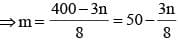∵ m and n are positive integers, so n must be a multiple of 8. i.e. n = 8, 16, 24, ....., 128, then we get m = 47, 44, 41, ....,2 respectively.
So, the number of ordered pairs (m, n) is 16.

## Topic-wise Past Year Questions for CAT

31 tests
 Use Code STAYHOME200 and get INR 200 additional OFF Use Coupon Code
Information about Test: Algebra - 1 Page
In this test you can find the Exam questions for Test: Algebra - 1 solved & explained in the simplest way possible. Besides giving Questions and answers for Test: Algebra - 1, EduRev gives you an ample number of Online tests for practice

## Topic-wise Past Year Questions for CAT

31 tests

### How to Prepare for CAT

Read our guide to prepare for CAT which is created by Toppers & the best Teachers